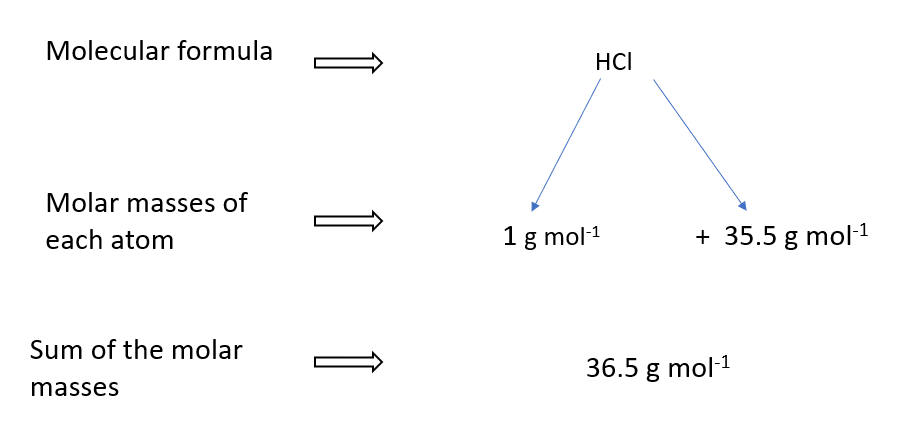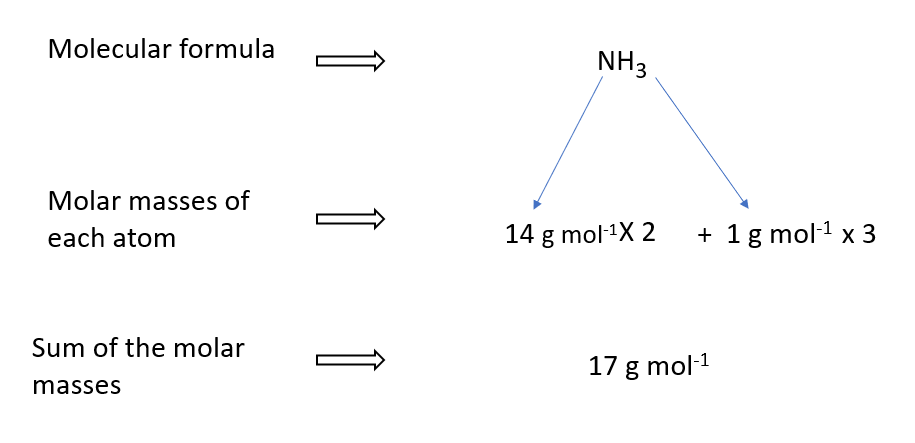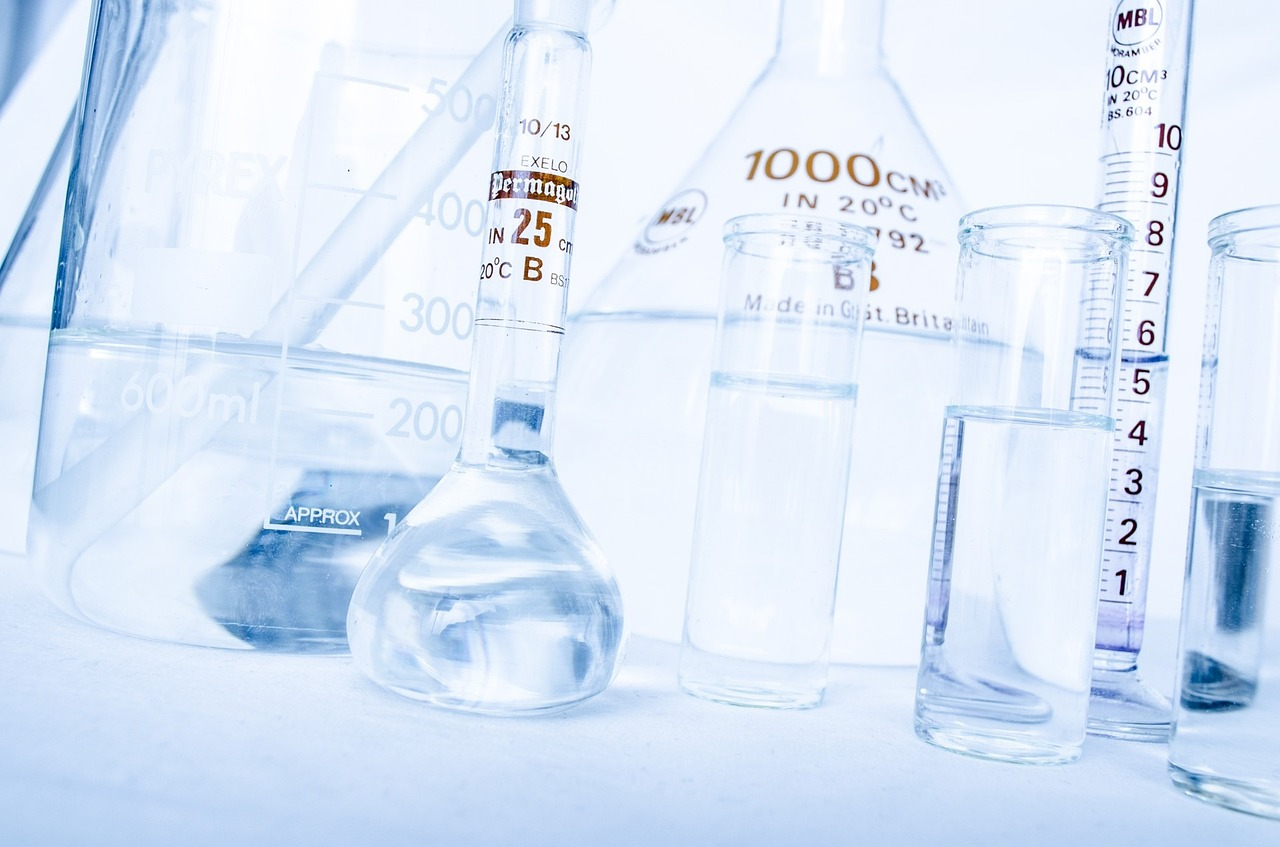# Calculate molar mass of compounds

## What is molar mass?

The mass of a mole of any substance is defined as its molar mass. Molar mass is measured in grams per mole (g mol-1) or kilograms per mole (kg mol-1).

## How to calculate molar mass of compounds?

The molar mass of an element or compound can be calculated if its molecular formula is known. This is because the molar mass of a compound equals the total of its atoms’ molar masses.

## Molar mass of several elements in a table

 Element Symbol Molar Mass (g/mol) Hydrogen H 1.008 Helium He 4.003 Lithium Li 6.941 Carbon C 12.011 Nitrogen N 14.007 Oxygen O 15.999 Fluorine F 18.998 Sodium Na 22.990 Magnesium Mg 24.305 Aluminum Al 26.982 Silicon Si 28.086 Chlorine Cl 35.453 Potassium K 39.098 Calcium Ca 40.078 Iron Fe 55.845 Copper Cu 63.546 Zinc Zn 65.38 Bromine Br 79.904 Silver Ag 107.868 Iodine I 126.904 Gold Au 196.967

## Molar mass of water

A water molecule, for example, is made up of two hydrogen atoms (H) and one oxygen atom (O). Water’s molar mass (H2O) is thus the sum of the molar masses of two hydrogen atoms and one oxygen atom.

Hydrogen has a relative atomic mass of one and oxygen has a relative atomic mass of sixteen, the molar mass of water can be computed as follows.## Molar mass of HCl## Molar mass of O2## Molar mass of NaCl## Molar mass of NaOH## Molar mass of ethanol

### steps to calculate the Molar mass of ethanol

• Identify the chemical formula for ethanol, which is C2H5OH.
• Find the atomic masses of each of the atoms in the formula. You can find these on the periodic table of elements.
• Carbon (C) has an atomic mass of 12.01 g/mol.
• Hydrogen (H) has an atomic mass of 1.008 g/mol.
• Oxygen (O) has an atomic mass of 15.999 g/mol.
• Multiply the atomic mass of each element by its atom count in the formula. In the case of ethanol:
• There are two carbon atoms in the formula, so the total mass contribution from carbon is 2 x 12.01 = 24.02 g/mol.
• There are six hydrogen atoms in the formula, so the total mass contribution from hydrogen is 6 x 1.008 = 6.048 g/mol.
• There is one oxygen atom in the formula, so the total mass contribution from oxygen is 1 x 15.999 = 15.999 g/mol.
• Add up the mass contributions of each element to get the molar mass of ethanol:
• Molar mass of ethanol = 24.02 g/mol (from carbon) + 6.048 g/mol (from hydrogen) + 15.999 g/mol (from oxygen) = 46.07 g/mol.

Therefore, the molar mass of ethanol is approximately 46.07 g/mol.

## How to calculate molar mass of CO2

To calculate the molar mass of CO2 (carbon dioxide), you need to add up the atomic masses of the elements that make up one molecule of CO2.

The formula for carbon dioxide is CO2, which means it has one carbon atom (C) and two oxygen atoms (O). To find the atomic masses, you can use the periodic table of elements.

The atomic mass of carbon (C) is 12.011 g/mol, and the atomic mass of oxygen (O) is 15.999 g/mol.

To calculate the molar mass of CO2, you simply add up the atomic masses of one carbon atom and two oxygen atoms:

Molar mass of CO2 = 1(C) x atomic mass of C + 2(O) x atomic mass of O

= 1(12.011 g/mol) + 2(15.999 g/mol)

= 44.01 g/mol

Therefore, the molar mass of CO2 is approximately 44.01 g/mol.

How to calculate NH3## Calculate molar mass of unknown acid titration

To calculate the molar mass of an unknown acid by titration, you need to perform an acid-base titration experiment using a standardized solution of a base, such as sodium hydroxide (NaOH), and an indicator to determine the endpoint of the titration.

### The general steps for performing the titration experiment are:

Weigh a known mass of the unknown acid and dissolve it in water to make a solution of known concentration.

Pipette a volume of the acid solution into a flask and add a few drops of indicator solution.

Titrate the acid solution by slowly adding the standardized solution of NaOH until the indicator changes color, indicating that the acid has been neutralized.

Record the volume of NaOH solution added to reach the endpoint of the titration.

Repeat the titration two more times to ensure accuracy and consistency of the results.

Once you have the data from the titration experiment, you can use the following formula to calculate the molar mass of the unknown acid:

Molar mass of acid = (Volume of NaOH x Molarity of NaOH x Molar mass of NaOH) / Volume of acid

In this formula, the volume of NaOH is the total volume of the NaOH solution used to titrate the acid, the molarity of NaOH is the concentration of the NaOH solution, the molar mass of NaOH is the molar mass of sodium hydroxide (40.00 g/mol), and the volume of acid is the volume of the unknown acid solution used in the titration.

By plugging in the appropriate values and solving for the molar mass of the unknown acid, you can determine its molar mass.